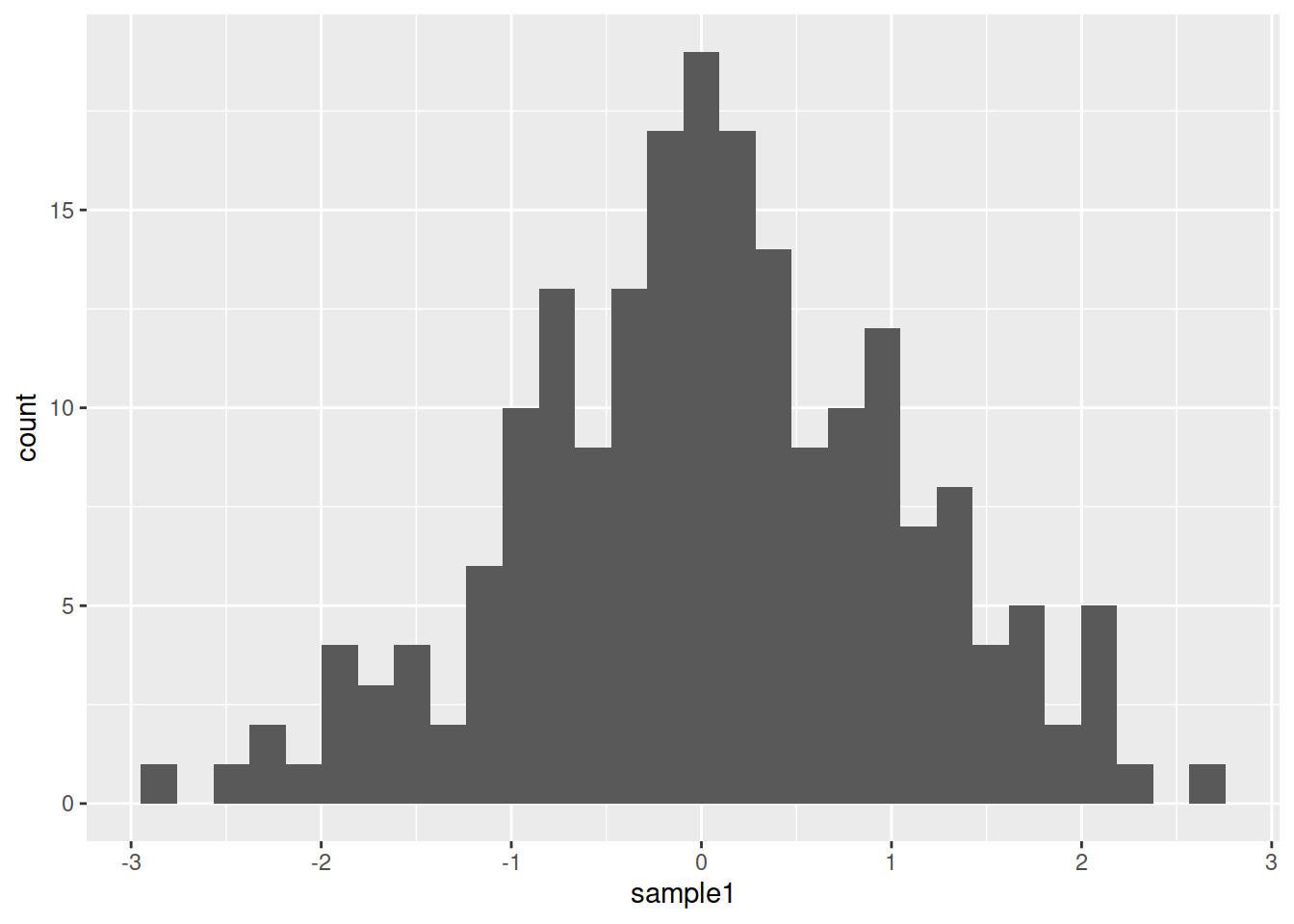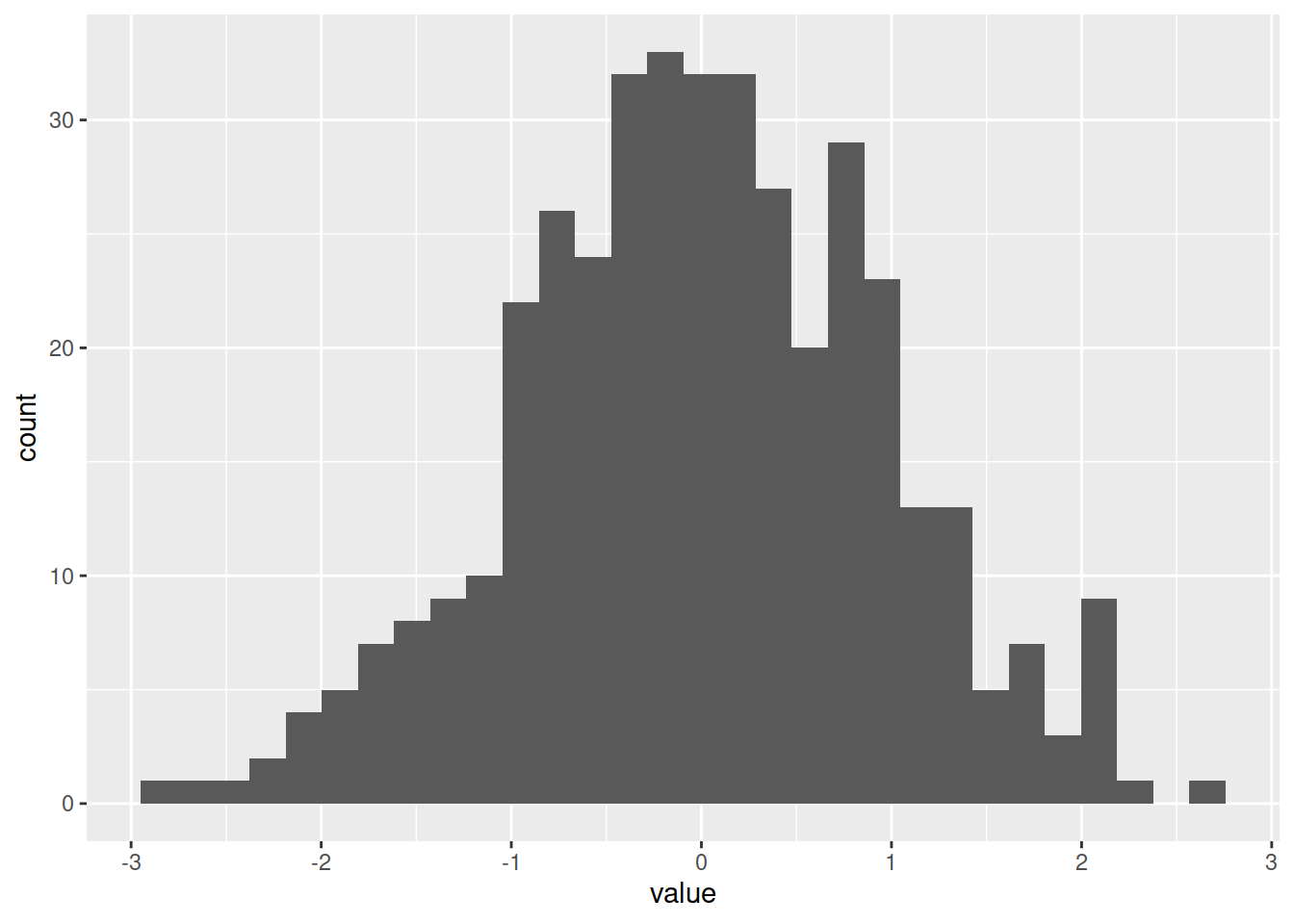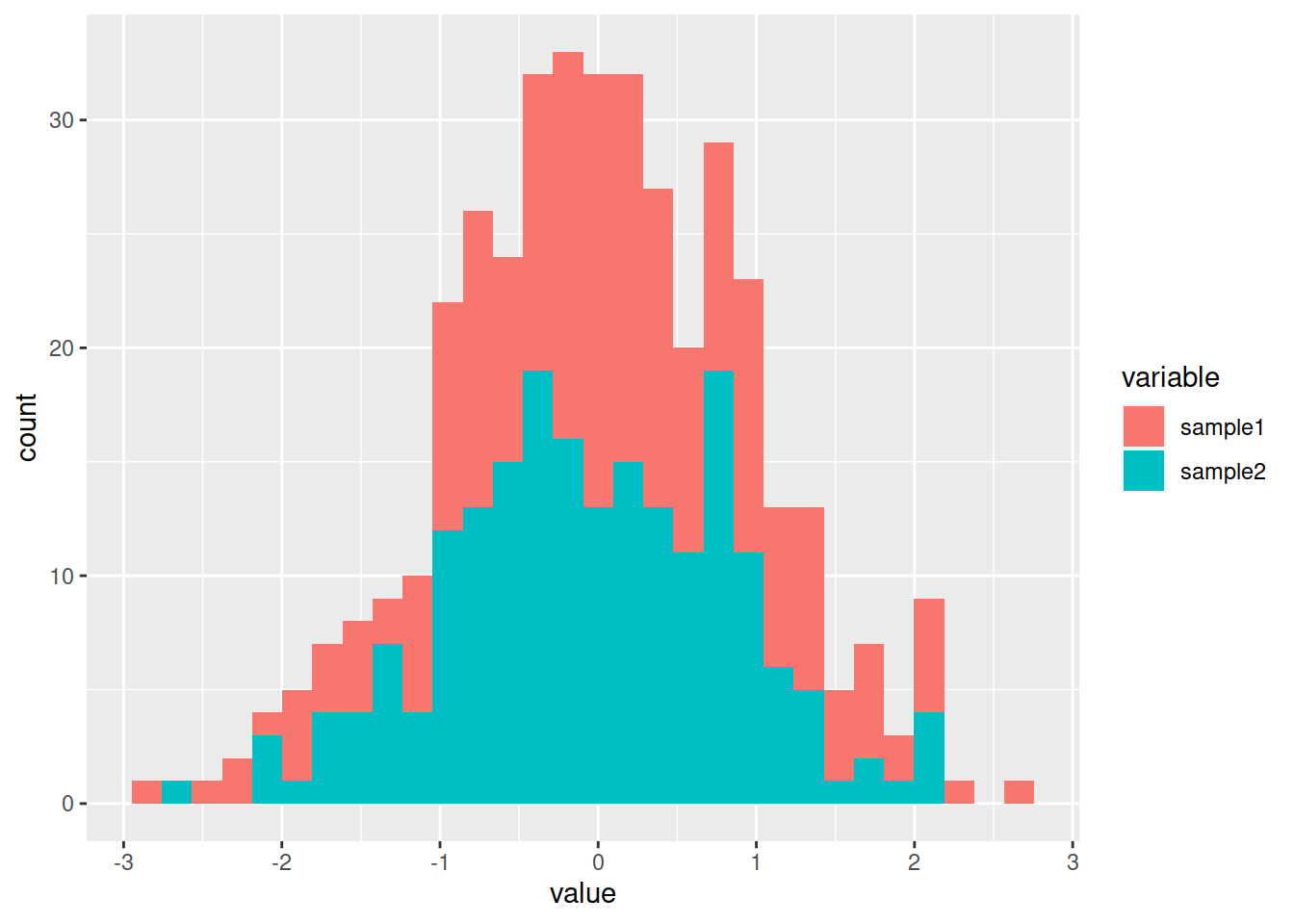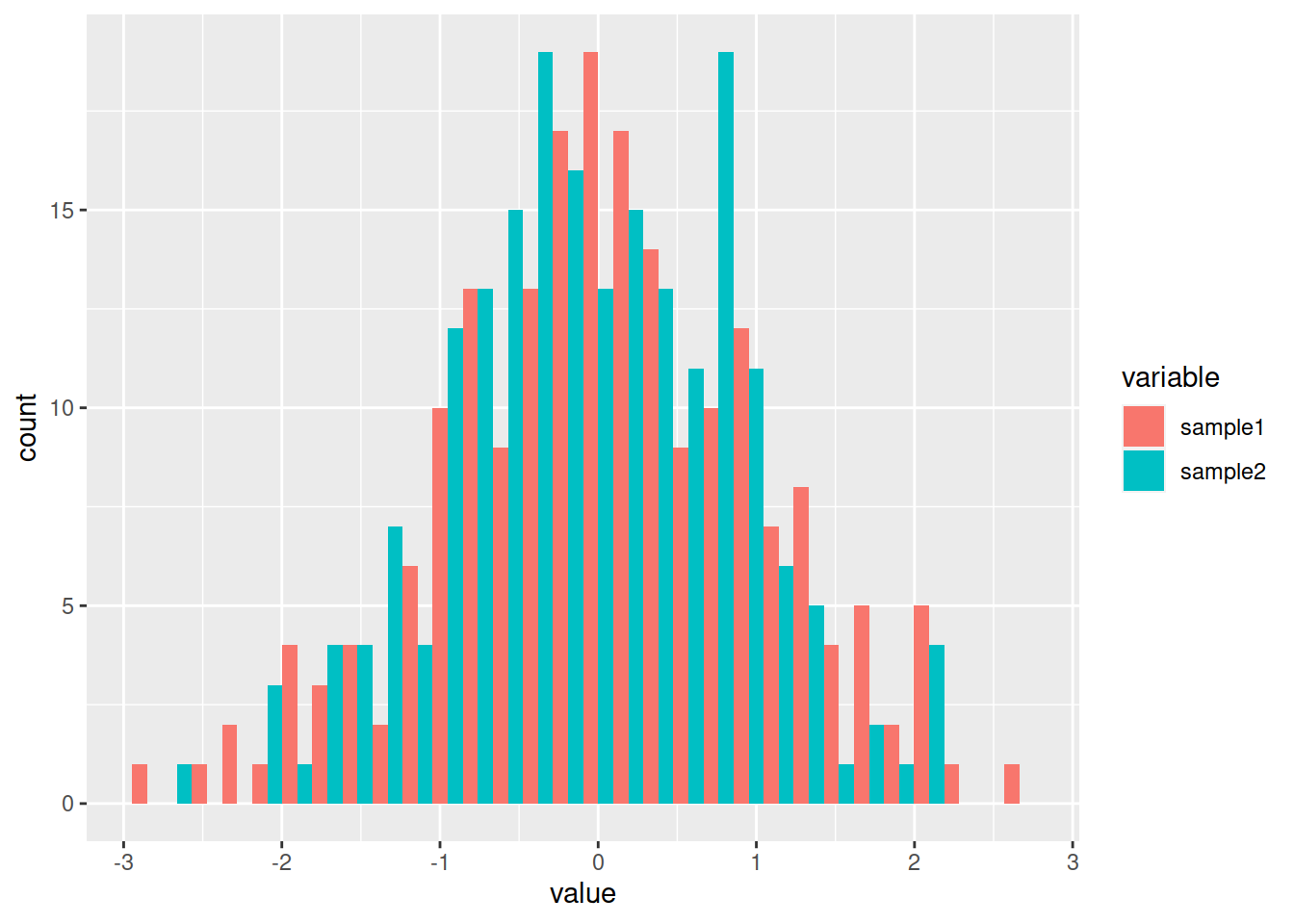## 16.5 Histograms

Simple histogram on one sample (using the df2 data frame):

``ggplot(data=df1, mapping=aes(x=sample1)) + geom_histogram()``
``## `stat_bin()` using `bins = 30`. Pick better value with `binwidth`.``Histogram on more samples (using df_long):

``ggplot(data=df_long, mapping=aes(x=value)) + geom_histogram()``
``## `stat_bin()` using `bins = 30`. Pick better value with `binwidth`.``Split the data per sample (“variable” column that represents here the samples):

``ggplot(data=df_long, mapping=aes(x=value, fill=variable)) + geom_histogram()``
``## `stat_bin()` using `bins = 30`. Pick better value with `binwidth`.``By default, the histograms are stacked: change to position dodge (side by side):

``````phist <- ggplot(data=df_long, mapping=aes(x=value, fill=variable)) +
geom_histogram(position='dodge')
phist``````
``## `stat_bin()` using `bins = 30`. Pick better value with `binwidth`.``HANDS-ON

Going back to the rock dataset:

• Create a histogram of the rocks perimeter.
• Add a density plot to the histogram, following instructions from this post
``````# Create a histogram of the rocks **perimeter**.